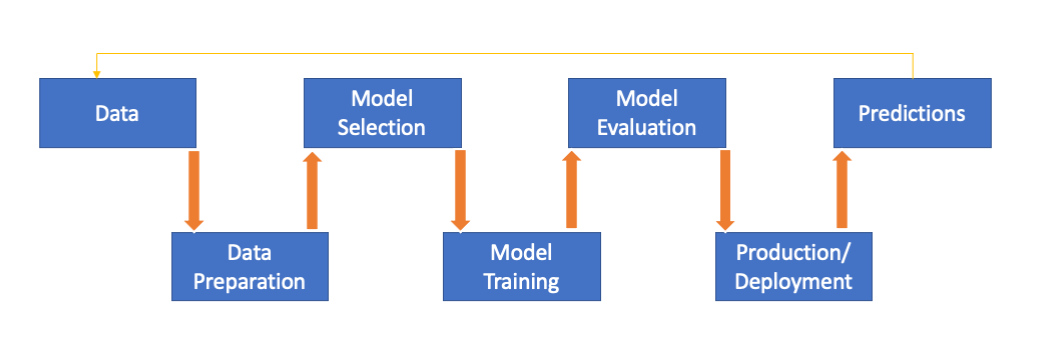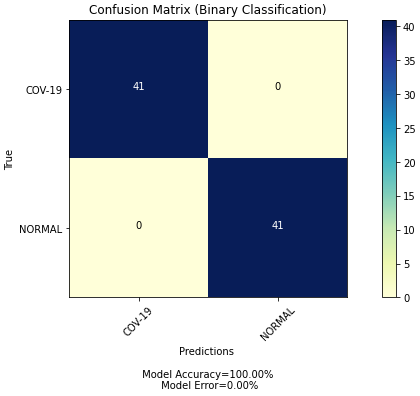# Confusion Matrix — Is it really confusing?

The most critical and essential part of any machine learning model building is the phase of “Model Evaluation”. On average, a Data Scientist spends 60% of their time cleaning and organizing data to ensure better prediction power and performance rate of a model. But how do we know if our model is effective? How do we calculate the performance of the developed model?Fig. 1: Machine Learning process flow

We need some evaluation metrics to measure performance and effectiveness. One such metric would be the Confusion Matrix. There are many classification metrics out there but we are more interested in the confusion matrix for now.

Okay, we now know the Confusion matrix is a metric, but what exactly is it? Let’s start with a basic definition.

Confusion Matrix, also know as an error matrix, is a specific table that is often used to describe the performance of a classification model on a set of test data for which the true values are known.

For example, the table below shows a confusion matrix of a two-class classification problem. The model here is detecting Covid positive patients via chest X-rays with 100% accuracy.Fig. 3: Confusion Matrix of Covid positive and Normal patients

You are like, “Great! I now know what a confusion matrix is, how it looks. But, how do I read it?”

Well, let us start with the four outputs in our Confusion Matrix:

1.True Positive (TP): It simply means that your prediction is positive and it’s true.

Recall our example from above, the model predicted that 41 subjects out of 82 subjects are Covid positive and that is true.

2.True Negative (TN): It means we predicted no and they don’t have the disease.

The model predicted the rest of the 41 subjects of 82 subjects don’t have Covid, hence they are Normal.

3.False Positive (FP): It means we predicted yes, but they don’t have the disease. This is also known as a “Type I error”.

In our case, the model was performing well so we don’t have a type I error. But let’s say the model predicted a False positive for a patient, this could cause serious complications. The patient is subjected to more tests and sometimes it could lead to an unnecessary financial and mental burden on both the patient and medical fraternity.

The consequences of making a type I error means that changes or interventions are made which are unnecessary, and thus waste time, resources, etc.

4.False Negative (FN): It means we predicted no, but they have the disease. This is also known as “Type II error”.

We do not have a Type II error in our case but if our model predicted a False Negative in the case of Covid, this would have serious implication not just for the patient but also for the others since now he might unknowingly pass it to people he might come in contact with. These have much worse implications because sometimes they don’t get the medical care they need to stop any further complications.

The consequences of making a type II error leads to the preservation of the status quo (i.e interventions remain the same) when change is needed.

There’s more to a Confusion Matrix than the four important concepts we discussed above. These are also sometimes referred to as advanced classification metrics and are mathematically expressed.

Sensitivity, also known as Recall or True Positive Rate is a measure of true positives that are correctly identified.

Referring back to our model, it is nothing but the proportion of patients that actually had COVID and were predicted true by the model. And Of course, we want this to be higher as high as possible.

Specificity, also known as True Negative Rate is a measure of true negatives that are correctly identified.

We had 41 True Negatives in our cases and 0 False Positives, the specificity is at 100%. Similar to Sensitivity, we want this number to be higher as well.

Precision is a ratio of the number of true positives to the number of true positives plus the number of false positives.

Based on our example, the precision is 100% since our False Positives are 0.

Accuracy is simply the fraction of the total sample that is currently identified.

The model above has 100% accuracy. But let’s put the numbers in the mathematical expression in order for validation.

F1 Score is the harmonic mean of precision and sensitivity. It’s a measure of test’s accuracy.

F1 score is a good choice when you seek to balance between Precision and Recall.

# Leveraging Python and scikit- earn library for the Confusion matrix

While working on the Chest X-ray classification problem for Covid positive patients, I had an opportunity to use the sci-kit learn library in order to generate a confusion matrix for analyzing my model's performance.

The complete code and project information is available on Github

# Evaluating the model and the classification report

In my experience, the confusion matrix is one among many great metrics available at hand to evaluate our classification models. As a Data Scientist, it is an essential skill to understand a confusion matrix and interpret a classification report.

I hope I provided you with a basic understanding of these concepts and showed you how you can leverage these to evaluate your own models. If you have any questions, please reach out to me. If you like the article and want to share it, please feel free!

--

--